# Electric Power

Voltage and current are two basic parameters of an electric circuit. But, only voltage and current are not sufficient to express the behaviour an electric circuit element. We essentially need to know, how much electric power, a circuit element can handle. All of us have seen that a 60 watts electric lamp gives less light than a 100 watts electric lamp. When we pay electric bill for electricity consumption, we are actually paying the cahrges for electric power for a specified period of time. Thus electric power calculation is quite essential for analyzing an electric circuit or network.

Power is the rate of energy supplied or consumed by an electric element with respect to time.

Suppose, an element supplies or consumes an energy of dw joules for a time of dt second, then power of the element can be represented as,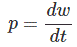This equation can also be rewritten as,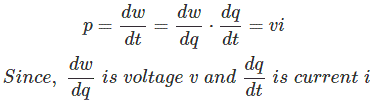Hence, from the as the expression of voltage and current in the equation are instantaneous, the power is also instantaneous. The expressed power is time-varying.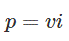So, the power of a circuit element is the product of voltage across the element and current through it.

As we have already told that a circuit element can either absorb or deliver power. We represent the absorption of power by putting a positive sign (+) in the expression of power. Likewise, we put a negative sign (-) when we represent the power delivered by the circuit element.

### Passive Sign Convention

There is a simple relationship between the direction of current, polarity of voltage and sign of the power of a circuit element. We call this simple relationship as passive sign convention. When a current enters in an element through its terminal of positive voltage polarity, we put a positive sign (+) before the product of the voltage and current. This implies that the element absorbs or consumes power from the electric circuit. On the other hand, when the current through the element leaves its terminal of positive voltage polarity, we put a negative sign (-) before the product of the voltage and current. This implies that the element delivers or supplies power to the electric circuit.

Let us take a resistor connected across two circuit terminals. Although, the rest of the circuit is not shown here in the figure. The polarity of the voltage drop across the resistor and the direction of current through the resistor are shown in the figure below. The resistor is consuming a power of vi watts as current i ampere enters in the resistor though its positive side of the dropped voltage v volt, as shown.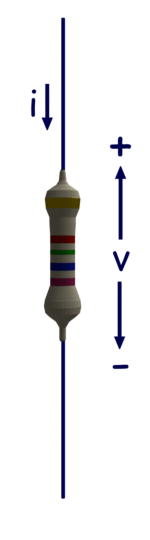Let us take a battery connected across two circuit terminals. Although, the rest of the circuit is not shown here in the figure. The polarity of the voltage drop across the battery and the direction of current through the battery are shown in the figure below. The battery is delivering a power of vi watts as current i ampere enters in the battery of v volt through its positive polarity terminal as shown.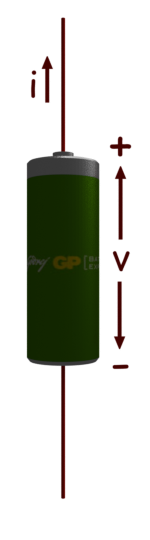Want To Learn Faster? 🎓
Get electrical articles delivered to your inbox every week.
No credit card required—it’s 100% free.